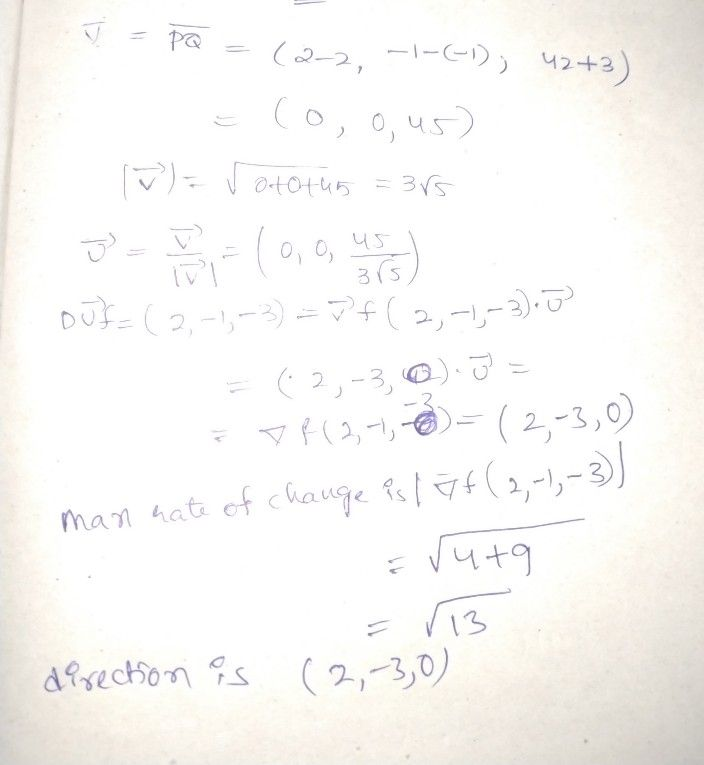Symbol
Problemb) $\right)$ Find the directional derivative of $y\left(x,y,=\right)=xy^{3}+yz^{3}$ at the point $\left(2,-1,-3\right)$ in the direction of vector $2i$ $-3\bar{i} +42\bar{k}$ $\left(6M\right)$
Calculus
Search count: 107
SolutionQanda teacher - harshitany doubt pls do ask me